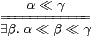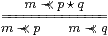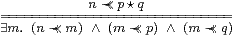## 11  The way-below relation

We now introduce the last (g) of our abstract characterisations of local compactness in the Introduction. Recall from Section 2 that any continuous distributive lattice carries a binary relation (written ≪ and called “way-below”) such that

 ⊥≪γNotation 11.1 We define a new relation n≺≺ m as Anβm.

This is an open binary relation (term of type Σ with two free variables) on the overt discrete object N of indices of a basis, not on the lattice ΣX. It is an “imposed” structure on N in the sense of Remark 4.5.

For most of the results of this section, we shall require (βn,An) to be an ∨-basis for X.

Examples 11.2 (Not all of these are ∨-bases.)

1. Let βn classify UnX, and An=λφ.(Kn⊂φ) in a locally compact sober space. Then n≺≺ m means that KnUm. This is consistent with Notation 1.3 if we identify the basis element n with the pair (UnKn).
2. Let An=λφ.(βn≪φ) in a continuous lattice. Then n≺≺ m means that βn≪βm, cf. Definition 2.3.
3. In the interval basis on ℝ in Example 6.10, <q,δ>≺≺<p,є> means that [q,δ]⊂(p,є), i.ep−є< q−δ≤ q+δ< p+є.
4. In the basis of disjoint pairs of opens, (UnVn), for a compact Hausdorff space in Example 6.11, n≺≺ m means that VnUm=X.
5. In the prime basis ({n},η n) for N in Example 1, n≺≺ m just when n=m.
6. In the Fin(N)-indexed filter ∨-basis on N in Proposition 8.8, ℓ≺≺ℓ′ iff ℓ⊂ℓ′.
7. In the prime ∧-basis on ΣN in Example 2, on the other hand, ℓ≺≺ℓ′ iff ℓ′⊂ℓ.
8. More generally, in the prime ∧-basis on ΣX derived from an ∨-basis on X in Lemma 9.8, (n≺≺ΣXm) iff (m≺≺X n).
9. In the Fin(Fin(N))-indexed filter lattice basis on ΣN in Proposition 8.8,
 L≺≺ R  ≡  AL BR  ⇔  R⊂♯L  ≡  ∀ℓ∈ L.∃ℓ′∈ R.(ℓ′⊂ℓ),
where RL is known as the upper order on subsets induced by the relation ⊂ on elements.
10. In the prime ∧-basis on ΣΣN in Lemma 8.9, L≺≺ R iff LR.
11. In the ∨-basis on ΣΣX derived from an ∨-basis on X in Lemma 9.9,
 (ℓ≺≺Σ2 Xℓ′)  ⇔ (ℓ≺≺X♯ℓ′).
12. Any stably locally compact object X has a filter lattice basis (N,0,1,+,⋆,≺≺) such that the opposite (N,1,0,⋆,+,≻≻) is the basis of another such object, known as its Lawson dual (Proposition 12.14).

Our first result just restates the assumption of an ∨-basis, cf. Lemmas 1.9 and 2.6:

Lemma 11.3 0≺≺ p, whilst n+m≺≺ p iff both n≺≺ p and m≺≺ p.

Proof    A0βp⇔⊤ and An+mβpAnβpAmβp.         ▫

In a continuous lattice, α≪β implies α≤β, but we have no similar property relating ≺≺ to ≼. We shall see the reasons for this in Section 13. But we do have two properties that carry most of the force of α≪β⇒α≤β. We call them roundedness. The second also incorporates many of the uses of directed joins and Scott continuity into a notation that will become increasingly more like discrete mathematics than it resembles the technology of traditional topology.

Lemma 11.4 βn=∃ m.(m≺≺ n)∧βm and Am=∃ n.An∧(m≺≺ n).

Proof    The first is simply the basis expansion of βn. For the second, we apply Am to the basis expansion of φ, so

 Amφ  ⇔  ∃ n.Anφ∧ Amβn  ⇔  (∃ n.An∧ Amβn)φ,

since An preserves directed joins (Theorem 9.6).         ▫

Corollary 11.5 If m≺≺ n then βm≤βn and AmAn.         ▫

Corollary 11.6 If m≺≺ n, AmAm and βn≤βn, then m′≺≺ n′.

Proof    (m≺≺ n)≡ AmβnAmβn≡(m′≺≺ n′).         ▫

Corollary 11.7 The relation ≺≺ satisfies transitivity and the interpolation lemma:

 (m≺≺ n)  ⇔  (∃ k. m≺≺ k≺≺ n).

Proof    Amβn ⇔ (∃ k.Akm≺≺ kn ⇔ ∃ k.Akβnm≺≺ k.         ▫

Now we consider the interaction between ≺≺ and the lattice structures (⊤,⊥,∧,∨) and (1,0,+,⋆). Of course, for this we need a lattice basis.

Lemma 11.8 As directed joins,

 φ∧ψ  =  ∃ p q.βp⋆ q∧ Apφ∧ Aqψ   and   φ∨ψ = ∃ p q.βp+q∧ Apφ∧ Aqψ.

Proof    The first is the Frobenius law, since βpqp∧βq.

The second uses Lemma 4.17: we obtain the expression

 φ∨ψ  =  ∃ p.Apφ∧(βp∨ψ)

from the basis expansion φ=∃ p.Apφ∧βp by adding ψ to the 0th term (since A0φ⇔⊤ and β0=⊥) and, harmlessly, Apφ∧ψ to the other terms. Similarly,

 βp∨ψ  =  ∃ q.Aqψ∧(βp∨βq)  =  ∃ q.Aqψ∧βp+q.

The joins are directed because because A0φ∧ A0ψ⇔⊤ and

 (Ap1φ ∧ Aq1ψ)  ∧  (Ap2φ∧ Aq2ψ)  ⇔  (Ap1+p2φ∧ Aq1+q2ψ).                 ▫

Lemma 11.9 For a lattice basis, An⊤⇔(n≺≺1), An⊥⇔(n≺≺0) and

 An(φ∧ψ) ⇔ ∃ p q. (n≺≺ p ⋆  q)∧ Apφ∧ Aqψ An(φ∨ψ) ⇔ ∃ p q. (n≺≺ p+q)∧ Apφ∧ Aqψ

Proof    The first two are Anβ1 and Anβ0. The other two are ∃ p q.Anpq)∧ Apφ∧ Aqψ and ∃ p q.Anp+q)∧ Apφ∧ Aqψ, which are An applied to the directed joins in Lemma 11.8.         ▫

Proposition 11.10 The lattice basis (βn,An) is a filter basis iff 1≺≺1 andProof    (n≺≺1)⇔ Anβ1An⊤, but recall that n≺≺1 for all n iff 1≺≺1.

The displayed rule is AmβpAmβqAmβpqAmp∧βq). Given this, by the Frobenius law and Lemma 11.9,

 Amφ∧ Amψ ⇔ ∃ p q.Apφ∧ Aqψ∧ Amβp∧ Amβq ⇔ ∃ p q.Apφ∧ Aqψ∧ (m≺≺ p)∧ (m≺≺ q) ⇔ ∃ p q.Apφ∧ Aqψ∧ (m≺≺ p⋆ q) ⇔ Am(φ∧ψ)         ▫

If we don’t have a filter basis, we have to let n “slip” by n≺≺ m, cf. Lemma 2.8:

Lemma 11.11 For any lattice basis,Proof    Downwards, interpolate n≺≺ m≺≺ pqp,q, then m≺≺ p,q by monotonicity. Conversely, using Corollary 11.5, if Anβm⇔⊤ and βm≤βpq then Anβpq⇔⊤.         ▫

The corresponding result for ∨ is our version of the Wilker property, cf. Proposition 1.10 and Lemma 2.7.

Lemma 11.12Proof    Lemma 11.9 with φ≡βp and ψ≡βq.        ▫

We shall summarise these rules in Definition 14.1.

There are special results that we have in the cases of overt and compact objects. We already know that 1≺≺ 1 iff the object is compact (cf. Lemma 6.5), but the lattice dual characterisation of overtness cannot be 0≺≺ 0, as that always happens.

Lemma 11.13 If X is overt then

 (n≺≺ m)  ⇒  (n≺≺ 0) ∨ (∃ y.βm y)   but   (n≺≺ 0)∧(∃ x.βn x)⇔⊥.

Proof    φ x⇒ ∃ yy so, using the Phoa principle (Axiom 3.6),

 Anφ  ⇒  An(λ x.∃ y.φ y)   ⇔  An⊥∨∃ y.φ y∧ An⊤  ⇒  (n≺≺ 0) ∨ ∃ y.φ y.

Putting φ≡βm,

 (n≺≺ m)  ≡  Anβm  ⇒  (n≺≺ 0)∨ ∃ y.βm y,

whilst (n≺≺ 0) ∧ ∃ xn x  ⇔  ∃ x.Anβ0∧βn x  ⇒  ∃ x.∃ n.An⊥∧βn x  ⇔  ∃ x.⊥ x  ⇔  ⊥.         ▫

By a similar argument, we have Johnstone’s “Townsend–Thoresen Lemma” [Joh84],

 (n≺≺ p+q) ⇒ (n≺≺ p) ∨ (∃ y.βq y).

Corollary 11.14 Given a compact overt object, it’s decidable whether it’s empty or inhabited, cf. Corollary 5.8.

Proof    As (1≺≺1)⇔⊤, the Lemma makes (1≺≺0) and ∃ x1 x≡∃ x.⊤ complementary.         ▫

Compact overt subobjects are, in fact, particularly well behaved in discrete [E] and Hausdorff [J] objects.

Sections 1316 are devoted to proving that the rules above for ≺≺ are complete, in the sense that from any abstract basis (N,0,1,+,⋆,≺≺) satisfying them we may recover an object of the category. This proof is very technical, so in the next section we consider a special case, in which the lattice structure plays a much lighter role.